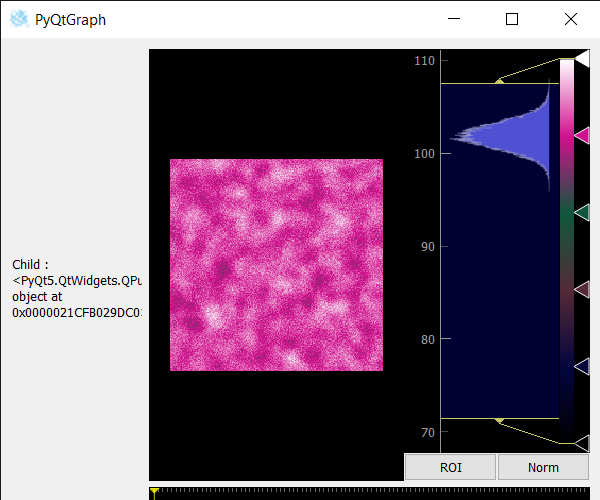Related Articles

# PyQtGraph – Getting Child of Image View at Given Position

• Last Updated : 24 Oct, 2020

In this article, we will see how we can get the child of the image view object at a given position in PyQTGaph. PyQtGraph is a graphics and user interface library for Python that provides functionality commonly required in designing and science applications. Its primary goals are to provide fast, interactive graphics for displaying data (plots, video, etc.). Widget used for display and analysis of image data. Implements many features like displaying 2D and 3D image data. For 3D data, a z-axis slider is displayed allowing the user to select which frame is displayed. Displays histogram of image data with a movable region defining the dark/light levels, editable gradient provides a color lookup table. Image view is a group of widget like it contains a view object, push-button and scroll bars.
We can create an image view with the help of the command given below

```# creating a pyqtgraph image view object
imv = pg.ImageView()
```

Syntax: ImageView(parent=None, name=’ImageView’, view=None, imageItem=None, levelMode=’mono’, *args)

Parameters:

• parent (QWidget): Specifies the parent widget to which this ImageView will belong. If None, then the ImageView is created with no parent.
• name (str): The name used to register both the internal ViewBox and the PlotItem used to display ROI data.
• view (ViewBox or PlotItem): If specified, this will be used as the display area that contains the displayed image.
• imageItem (ImageItem): If specified, this object will be used to display the image. Must be an instance of ImageItem or other compatible object.
• levelMode: specifies the *levelMode* argument

Returns: Object of class ImageView

In order to do this, we use childAt() method with the image view object

Syntax : imv.childAt(x, y)
Argument : It takes two integers as argument
Return : It returns QWidget object

Below is the implementation

## Python3

 `# importing Qt widgets``from` `PyQt5.QtWidgets ``import` `*`` ` `# importing system``import` `sys`` ` `# importing numpy as np``import` `numpy as np`` ` `# importing pyqtgraph as pg``import` `pyqtgraph as pg``from` `PyQt5.QtGui ``import` `*``from` `PyQt5.QtCore ``import` `*`` ` `# Image View class``class` `ImageView(pg.ImageView):`` ` `    ``# constructor which inherit original``    ``# ImageView``    ``def` `__init__(``self``, ``*``args, ``*``*``kwargs):``        ``pg.ImageView.__init__(``self``, ``*``args, ``*``*``kwargs)`` ` `class` `Window(QMainWindow):`` ` `    ``def` `__init__(``self``):``        ``super``().__init__()`` ` `        ``# setting title``        ``self``.setWindowTitle(``"PyQtGraph"``)`` ` `        ``# setting geometry``        ``self``.setGeometry(``100``, ``100``, ``600``, ``500``)`` ` ` ` `        ``# icon``        ``icon ``=` `QIcon(``"skin.png"``)`` ` `        ``# setting icon to the window``        ``self``.setWindowIcon(icon)`` ` `        ``# calling method``        ``self``.UiComponents()`` ` `        ``# showing all the widgets``        ``self``.show()`` ` `    ``# method for components``    ``def` `UiComponents(``self``):`` ` `        ``# creating a widget object``        ``widget ``=` `QWidget()`` ` `        ``# creating a label``        ``label ``=` `QLabel(``"Geeksforgeeks Image View"``)`` ` `        ``# setting minimum width``        ``label.setMinimumWidth(``130``)`` ` `        ``# making label do word wrap``        ``label.setWordWrap(``True``)`` ` `        ``# setting configuration options``        ``pg.setConfigOptions(antialias ``=` `True``)`` ` `        ``# creating image view view object``        ``imv ``=` `ImageView()`` ` `        ``# Create random 3D data set with noisy signals``        ``img ``=` `pg.gaussianFilter(np.random.normal(size``=``(``200``, ``200``)), ``                                ``(``5``, ``5``)) ``*` `20` `+` `100`` ` `        ``# setting new axis to image``        ``img ``=` `img[np.newaxis, :, :]`` ` `        ``# decay data``        ``decay ``=` `np.exp(``-``np.linspace(``0``, ``0.3``, ``100``))[:, np.newaxis, np.newaxis]`` ` `        ``# random data``        ``data ``=` `np.random.normal(size``=``(``100``, ``200``, ``200``))``        ``data ``+``=` `img ``*` `decay``        ``data ``+``=` `2`` ` `        ``# adding time-varying signal``        ``sig ``=` `np.zeros(data.shape[``0``])``        ``sig[``30``:] ``+``=` `np.exp(``-``np.linspace(``1``, ``10``, ``70``))``        ``sig[``40``:] ``+``=` `np.exp(``-``np.linspace(``1``, ``10``, ``60``))``        ``sig[``70``:] ``+``=` `np.exp(``-``np.linspace(``1``, ``10``, ``30``))`` ` `        ``sig ``=` `sig[:, np.newaxis, np.newaxis] ``*` `3``        ``data[:, ``50``:``60``, ``30``:``40``] ``+``=` `sig`` ` `        ``# setting image to image view``        ``# Displaying the data and assign each frame a time value from 1.0 to 3.0``        ``imv.setImage(data, xvals``=``np.linspace(``1.``, ``3.``, data.shape[``0``]))`` ` `        ``## Set a custom color map``        ``colors ``=` `[``            ``(``0``, ``0``, ``0``),``            ``(``4``, ``5``, ``61``),``            ``(``84``, ``42``, ``55``),``            ``(``15``, ``87``, ``60``),``            ``(``208``, ``17``, ``141``),``            ``(``255``, ``255``, ``255``)``        ``]`` ` `        ``# color map``        ``cmap ``=` `pg.ColorMap(pos``=``np.linspace(``0.0``, ``1.0``, ``6``), color ``=` `colors)`` ` `        ``# setting color map to the image view``        ``imv.setColorMap(cmap)`` ` `        ``# Creating a grid layout``        ``layout ``=` `QGridLayout()`` ` `        ``# minimum width value of the label``        ``label.setFixedWidth(``130``)`` ` `        ``# setting this layout to the widget``        ``widget.setLayout(layout)`` ` `        ``# adding label in the layout``        ``layout.addWidget(label, ``1``, ``0``)`` ` `        ``# plot window goes on right side, spanning 3 rows``        ``layout.addWidget(imv, ``0``, ``1``, ``3``, ``1``)`` ` `        ``# setting this widget as central widget of the main widow``        ``self``.setCentralWidget(widget)`` ` `        ``# getting child of image view at 10, 10``        ``value ``=` `imv.childAt(``10``, ``10``)`` ` `        ``# setting text to the label``        ``label.setText(``"Child : "` `+` `str``(value))`` ` `# create pyqt5 app``App ``=` `QApplication(sys.argv)`` ` `# create the instance of our Window``window ``=` `Window()`` ` `# start the app``sys.exit(App.``exec``())`

Output :Attention geek! Strengthen your foundations with the Python Programming Foundation Course and learn the basics.

To begin with, your interview preparations Enhance your Data Structures concepts with the Python DS Course. And to begin with your Machine Learning Journey, join the Machine Learning – Basic Level Course

My Personal Notes arrow_drop_up